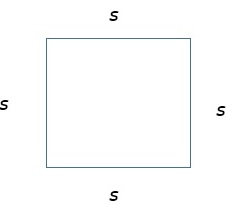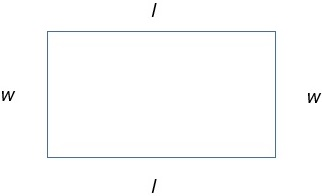# Perimeter of a Square or a Rectangle

#### Complete Python Prime Pack

9 Courses     2 eBooks

#### Artificial Intelligence & Machine Learning Prime Pack

6 Courses     1 eBooks

#### Java Prime Pack

9 Courses     2 eBooks

The perimeter of a square is the total length of the four equal sides of the square.

FormulaIf the side of a square is s, the perimeter of the square is

= 4 × s = 4 s

The perimeter of a rectangle is the total length of the two lengths and two widths of the rectangle.

FormulaIf the length and width of a rectangle are l and w, then its perimeter

= 2(l + w) units

If the side of a square is 8 m, find the perimeter of the square

### Solution

Step 1:

Side length of the square = 8 m

Step 2:

The perimeter of the square = 4 × 8 = 32 m

If the length and width of a rectangle are 8 and 4 units, then find its perimeter.

### Solution

Step 1:

Length and width of the rectangle are 8 and 4 units

Step 2:

Perimeter of rectangle

= 2 (l + w) = 2(8 + 4)

= 2 × 12 = 24 units